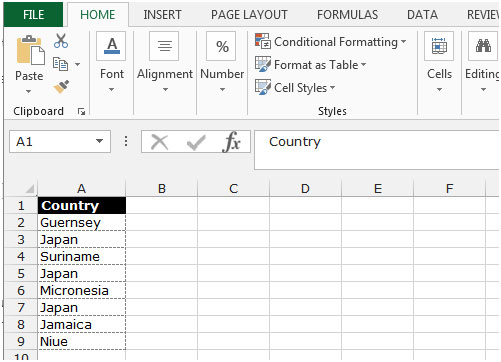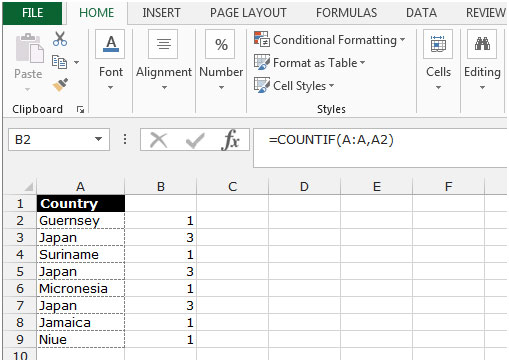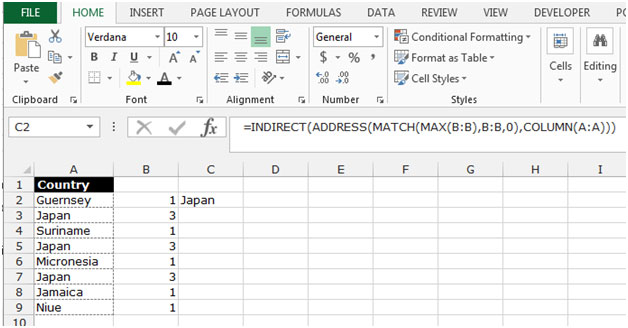# Find the most commonly repeated string in a column in Microsoft Excel 2010

In this article, we will learn how to find the most commonly repeated string in a column. We use the COUNTIF, INDIRECT, ADDRESS, MATCH, MAX ,COLUMN and MODE functions in Microsoft Excel.

COUNTIF function is used to count the duplicity of text or numbers in the range.

MATCH function we use to returns the cell number where the value is found in a horizontal or vertical range.

MAX function is used to return the largest number in a set of values. It ignores logical values and text.

COLUMN function is used to return the column number of a reference.

INDIRECT function returns the reference specified by a text string. This function is used to create a reference that won’t change if rows or columns are inserted on the worksheet.

ADDRESS function is used to create a cell reference as text, given specified row and column numbers.

Let’s take an example to understand how we can find the most commonly repeated string in a column in Microsoft Excel.

We have data in range A2:A9 in which column A contains the country name. Now, we want to check which country’s name is repeating the most.• Write the formula in cell B2.
• =COUNTIF(A:A,A2)
• Press Enter on your keyboard
• The function will give the count of every country,  Copy the same formula by pressing the key Ctrl+C and paste in the range by pressing the key Ctrl+V on your keyboard• Select the cell C1, and write the formula
• Press Enter on your keyboard
• The function will return the country’s name which is repeating more than othersThis is the way by which we can find the mostly repeated string in a column in Microsoft Excel.We would love to hear from you, do let us know how we can improve, complement or innovate our work and make it better for you. Write us at info@exceltip.com

1.This worked perfectly for me. Could you help with finding the 2nd most and 3rd most common string?

2.The excel tip has been corrected

3.